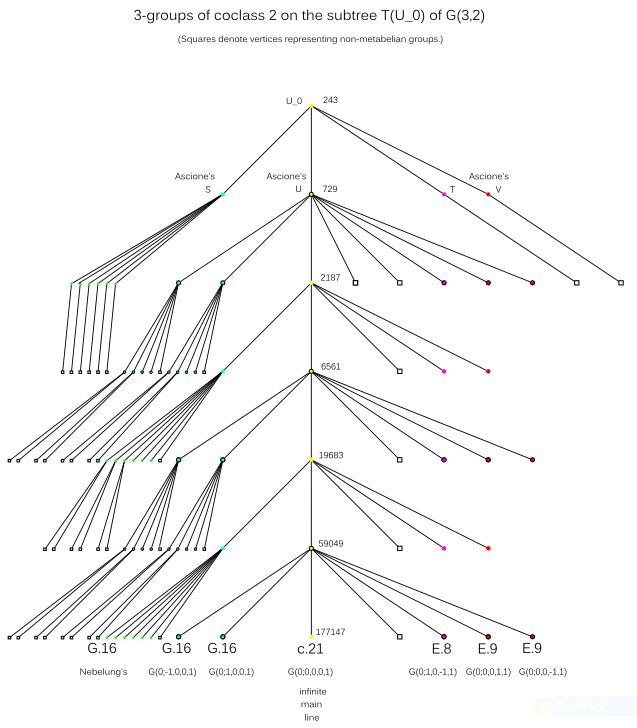# Oujda Research Project 2011

## The Subtree T(U0) of the Coclass Graph G(3,2)

The vertices of the coclass graph G(3,2) represent all finite 3-groups G of coclass cc(G)=2.
G(3,2) consists of finitely many sporadic groups and 16 coclass trees,
each with a single infinite main line and a structural periodicity.

The descendants of U0 form one of these trees, T(U0).
They are characterized by the isomorphism invariant ε = 0, indicating that
none of the abelianizations Mi/M'i (1 ≤ i ≤ 4)
of their maximal subgroups is of type (3,3,3).

Succinct presentation of structural details:
All capable vertices represent metabelian groups (circles).
However, several terminal vertices represent non-metabelian groups (squares).

With the aid of colors we characterize the transfer types:
the main line of type c.21 (0231) (yellow) and coclass families of types E.8 (1231) (magenta), E.9 (2231) (red),
and G.16 (4231) (cyan, with center of type (3,3), and green, with cyclic center of order 3).Applications in class field theory:
Only those vertices of T(U0) which are represented by circles with black contours
can be realized as second 3-class groups of real quadratic number fields K = Q(sqrt(D)),
that is, Galois groups Gal(F32(K)|K) of the second Hilbert 3-class field.
They are located at depth zero, one, and two on the odd branches of the tree T(U0).

 * Web master's e-mail address: contact@algebra.at *